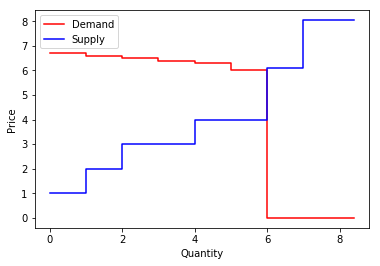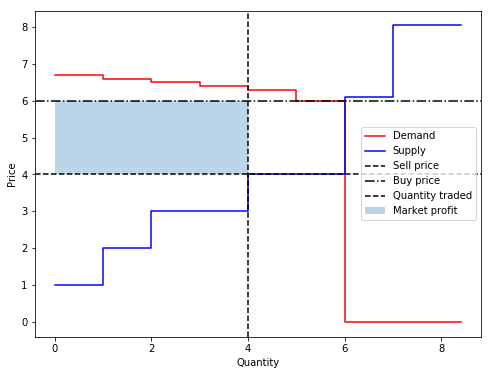# Huang¶

:

%matplotlib inline
import numpy as np
import pandas as pd
import matplotlib.pyplot as plt
import networkx as nx
import pymarket as pm


## Creates new market¶

:

mar = pm.Market()

mar.accept_bid(1, 6.7, 0, True, 0)
mar.accept_bid(1, 6.6, 1, True, 0)
mar.accept_bid(1, 6.5, 2, True, 0)
mar.accept_bid(1, 6.4, 3, True, 0)
mar.accept_bid(1, 6.3, 4, True, 0)
mar.accept_bid(1, 6, 5, True, 0)

mar.accept_bid(1, 1, 6, False, 0)
mar.accept_bid(1, 2, 7, False, 0)
mar.accept_bid(2, 3, 8, False, 0)
mar.accept_bid(2, 4, 9, False, 0)
mar.accept_bid(1, 6.1, 10, False, 0)

bids = mar.bm.get_df()
transactions, extras = mar.run('huang')
stats = mar.statistics()


## Orignal supply and demand curves¶

:

mar.plot()## Supply and demand curves after market is splitted¶

:

fig, ax = plt.subplots(figsize=(8, 6))
ax = mar.plot_method('huang', ax=ax)## Analysis of the trade¶

• Selling Price: 4, defined by bid 9, consequently, 9 does not trade
• Buying Price: 6, defined by bid 5, consequently, 5 does not trade

• Buying: 0, 1, 2, 3
• Selling: 6, 7, 8

### Results¶

• Supply and demand have the same size.
• The profit of the market maker coincides with the blue shaded area

## Statistics¶

:

print('Percentage of the maximum possible traded quantity')

Percentage of the maximum possible traded quantity

:

0.6666666666659999

:

print('Percentage of the maximum possible total welfare')
stats['percentage_welfare']

Percentage of the maximum possible total welfare

:

0.4186046511627907

:

print('Profits per user')
for u in bids.user.unique():
print(f'User {u:2} obtained a profit of {stats["profits"]["player_bid"][u]:0.2f}')

Profits per user
User  0 obtained a profit of 0.56
User  1 obtained a profit of 0.48
User  2 obtained a profit of 0.40
User  3 obtained a profit of 0.32
User  4 obtained a profit of 0.24
User  5 obtained a profit of 0.00
User  6 obtained a profit of 3.00
User  7 obtained a profit of 2.00
User  8 obtained a profit of 2.00
User  9 obtained a profit of 0.00
User 10 obtained a profit of 0.00

:

print(f'Profit to Market Maker was {stats["profits"]["market"]:0.2f}')

Profit to Market Maker was 8.00

[ ]: